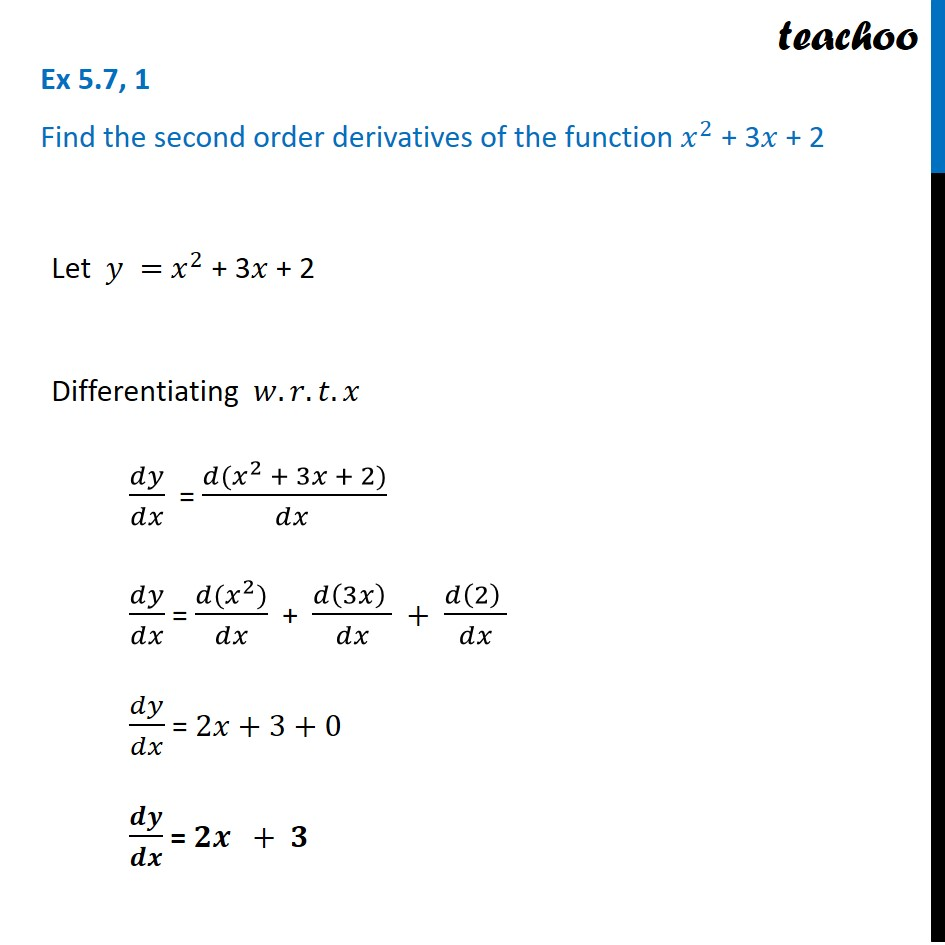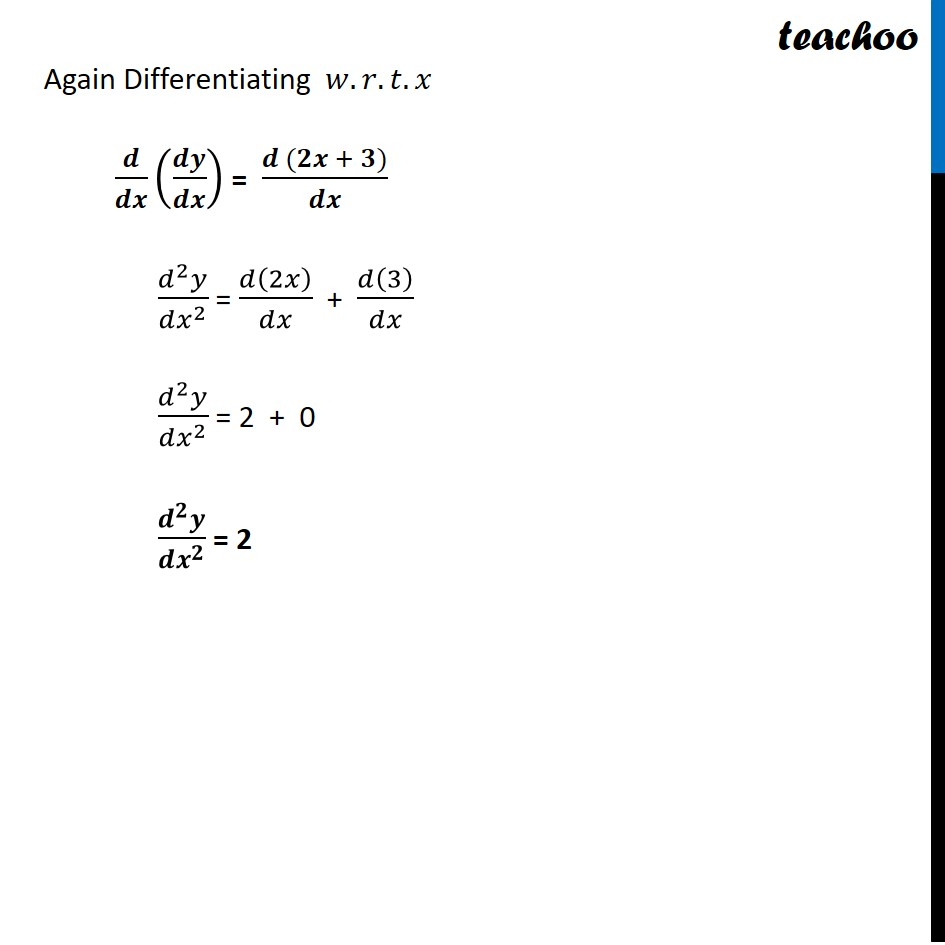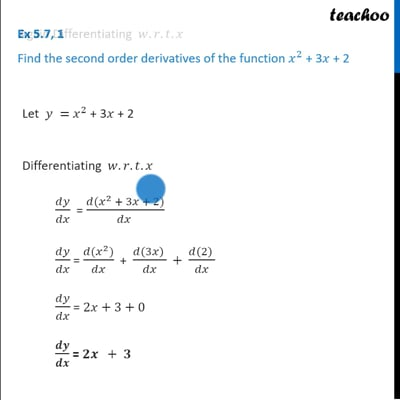Ex 5.7

Chapter 5 Class 12 Continuity and Differentiability
Serial order wiseThis video is only available for Teachoo black users

Introducing your new favourite teacher - Teachoo Black, at only ₹83 per month

### Transcript

Ex 5.7, 1 Find the second order derivatives of the function 𝑥^2 + 3𝑥 + 2 Let 𝑦 = 𝑥^2 + 3𝑥 + 2 Differentiating 𝑤.𝑟.𝑡.𝑥 𝑑𝑦/𝑑𝑥 = (𝑑(𝑥^2 + 3𝑥 + 2))/(𝑑𝑥 ) 𝑑𝑦/𝑑𝑥 = (𝑑(𝑥^2))/𝑑𝑥 + (𝑑(3𝑥) )/𝑑𝑥 + (𝑑(2) )/𝑑𝑥 𝑑𝑦/𝑑𝑥 = 2𝑥+3+0 𝒅𝒚/𝒅𝒙 = 𝟐𝒙 + 𝟑 Again Differentiating 𝑤.𝑟.𝑡.𝑥 𝒅/𝒅𝒙 (𝒅𝒚/𝒅𝒙) = (𝒅 (𝟐𝒙 + 𝟑))/𝒅𝒙 (𝑑^2 𝑦)/〖𝑑𝑥〗^2 = 𝑑(2𝑥)/𝑑𝑥 + 𝑑(3)/𝑑𝑥 (𝑑^2 𝑦)/〖𝑑𝑥〗^2 = 2 + 0 (𝒅^𝟐 𝒚)/〖𝒅𝒙〗^𝟐 = 2